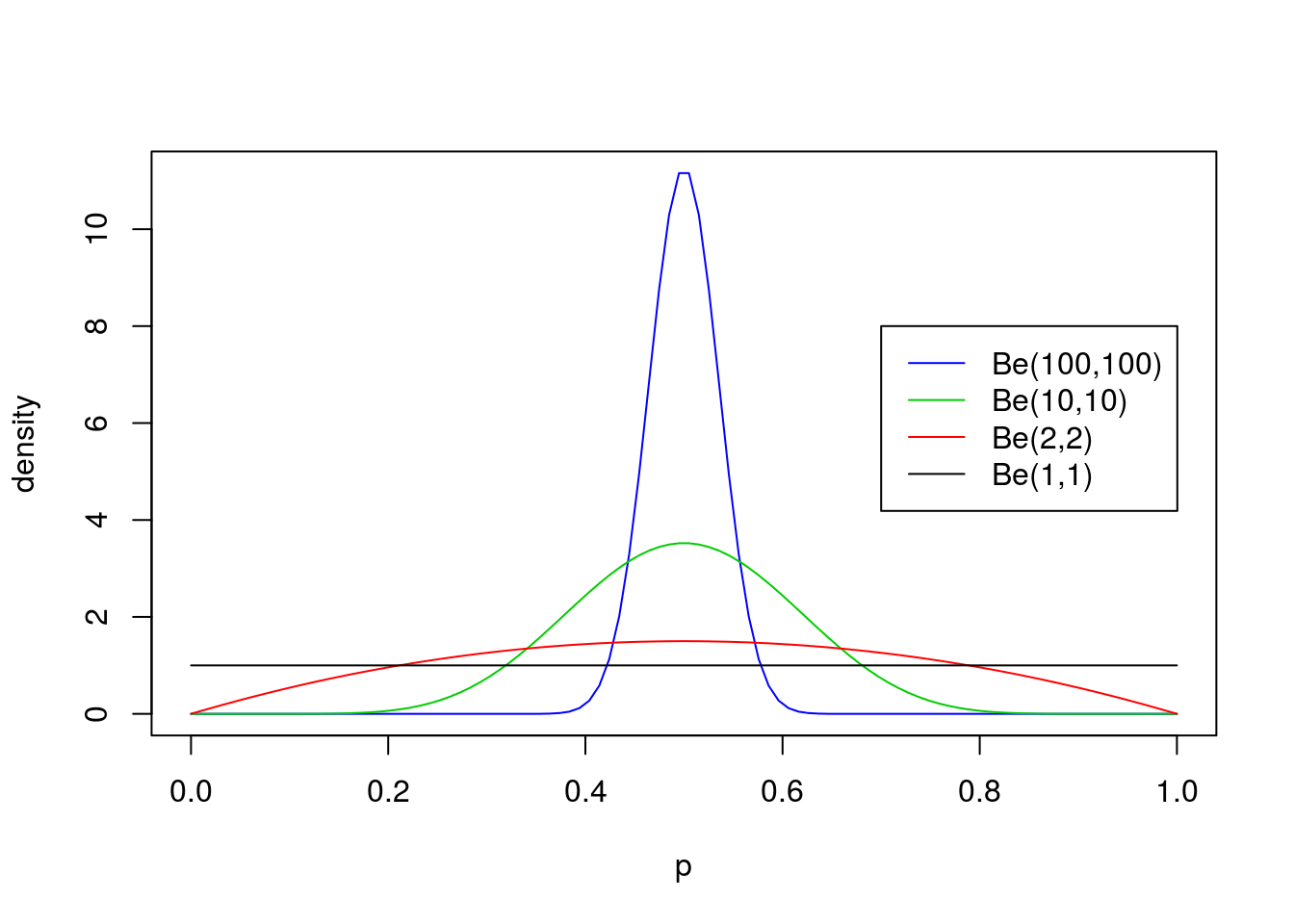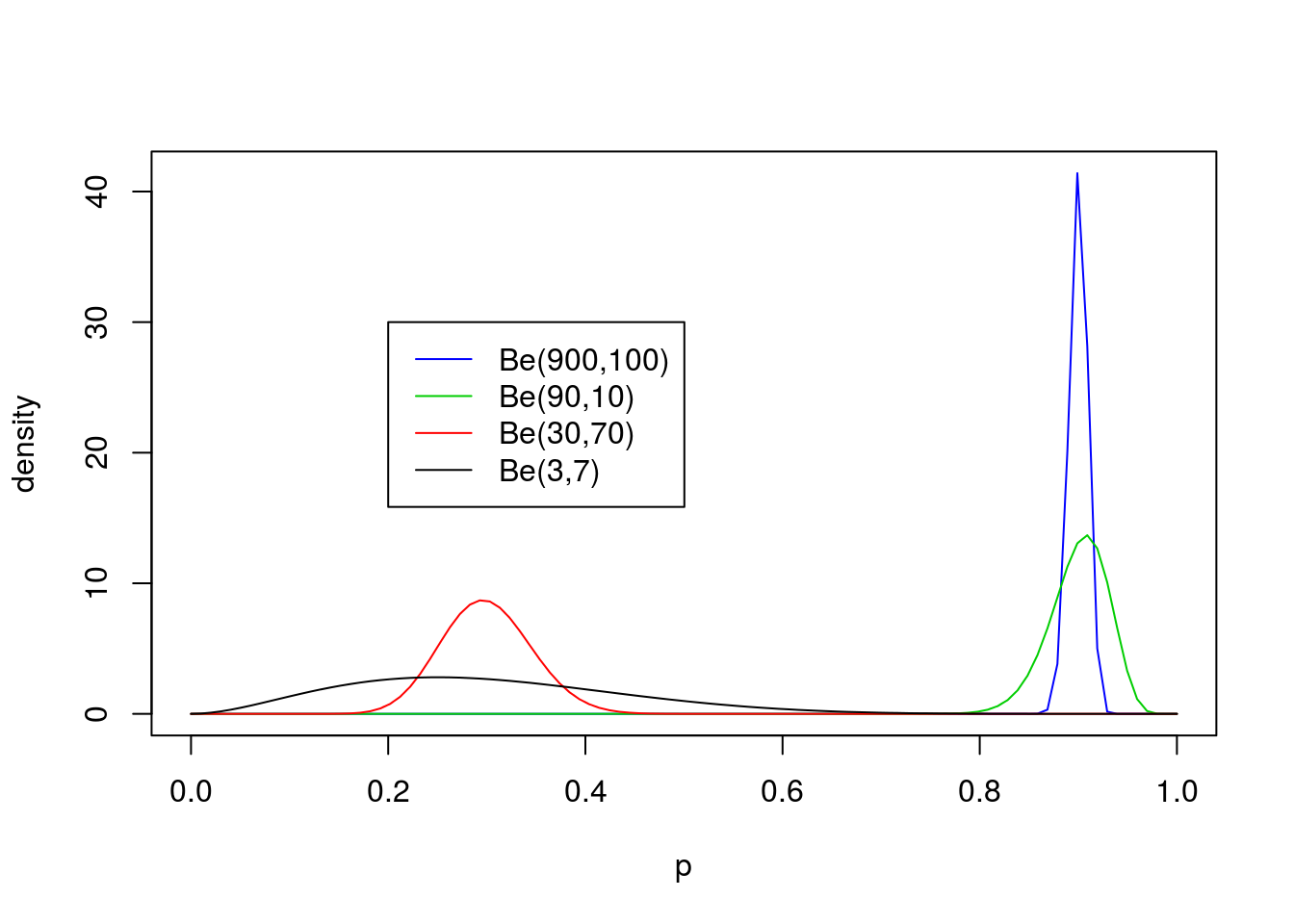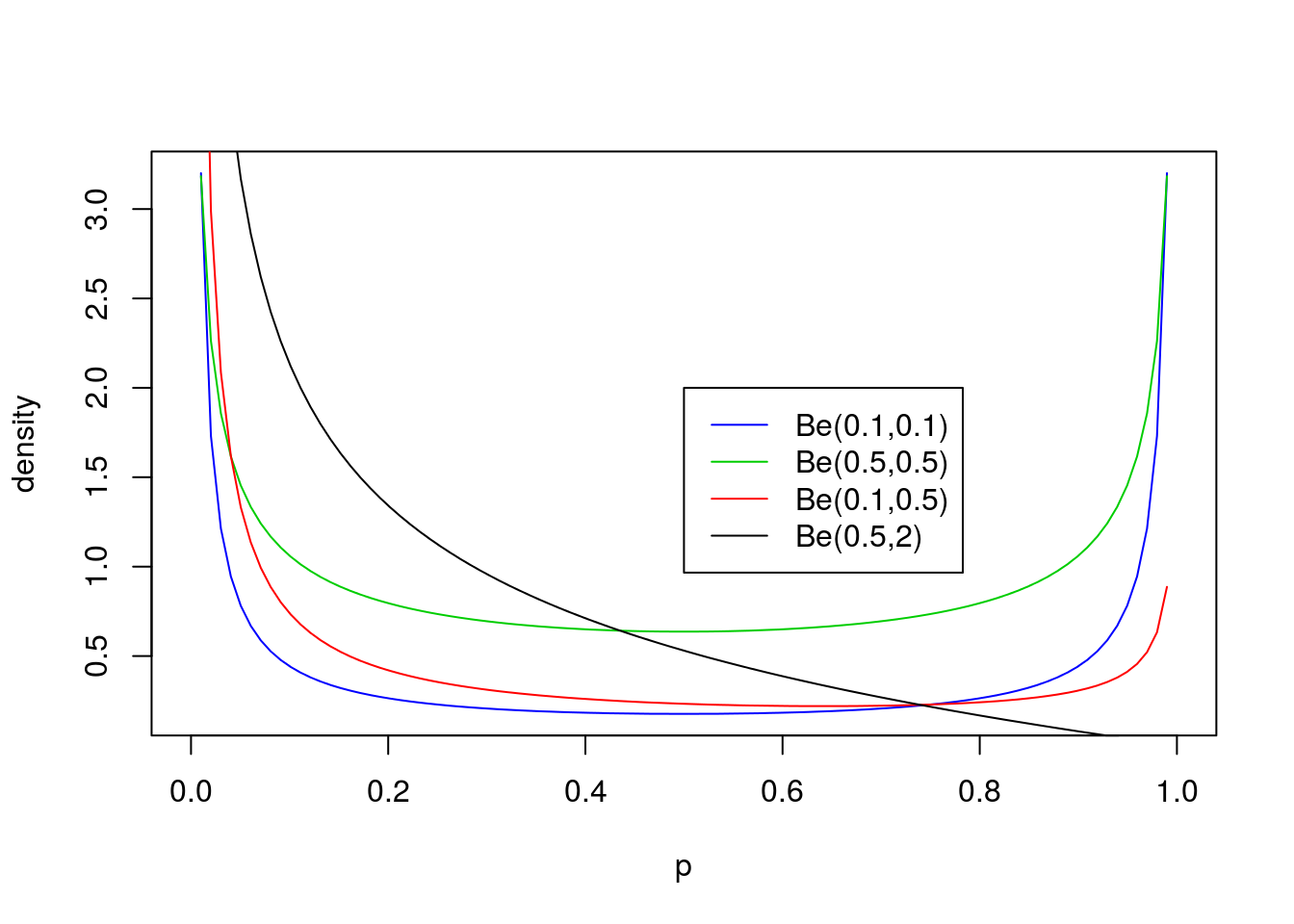Last updated: 2017-03-06

Code version: c7339fc

# Overview

The purpose of this vignette is to introduce the Beta distribution. You should be familiar with basic concepts related to distributions before - e.g. maybe you have come across the normal distribution and a uniform distribution before, and understand what it would mean to talk about their mean, variance and density.

If you want more details you could look at Wikipedia.

# The Beta Distribution

The Beta distribution is a distribution on the interval $$[0,1]$$. Probably you have come across the $$U[0,1]$$ distribution before: the uniform distribution on $$[0,1]$$. You can think of the Beta distribution as a generalization of this that allows for some simple non-uniform distributions for values between 0 and 1.

The Beta distribution has two parameters, which we will call $$a$$ and $$b$$. These two parameters determine the shape of the Beta distributions (just as the mean and variance determine the shape of the normal distribution).

Following the usual convention, we will write $$X \sim Be(a,b)$$ as shorthand for “$$X$$ has a Beta distribution with parameters $$a$$ and $$b$$”.

# Density

If $$X \sim Be(a,b)$$ then the density of $$X$$ is: $f_X(x) = \frac{1}{B(a,b)} x^{a-1}(1-x)^{b-1} \qquad (x \in [0,1]).$

For those of you that are interested, $$B(a,b)$$ is known as the “beta function” and is given by the integral $B(a,b) = \int_0^1 x^{a-1} (1-x)^{b-1} \,dx.$ This is where the beta distribution gets its name: its density involves the beta function. However, for this introduction you do not have to worry very much about what $$B(a,b)$$ is: think of it as a constant (in that it does not depend on $$x$$), that is included so that the density integrates to 1, as all densities must.

Because the Beta distribution is widely used, R has the built in function dbeta to compute this density. We will use this to look at some examples of the Beta distribution below.

# Examples

First we will look at some examples for $$a=b$$, with both $$\geq 1$$:

p = seq(0,1, length=100)
plot(p, dbeta(p, 100, 100), ylab="density", type ="l", col=4)
lines(p, dbeta(p, 10, 10), type ="l", col=3)
lines(p, dbeta(p, 2, 2), col=2)
lines(p, dbeta(p, 1, 1), col=1)
legend(0.7,8, c("Be(100,100)","Be(10,10)","Be(2,2)", "Be(1,1)"),lty=c(1,1,1,1),col=c(4,3,2,1))Now non-equal values of $$a$$ and $$b$$ with both $$\geq 1$$:

p = seq(0,1, length=100)
plot(p, dbeta(p, 900, 100), ylab="density", type ="l", col=4)
lines(p, dbeta(p, 90, 10), type ="l", col=3)
lines(p, dbeta(p, 30, 70), col=2)
lines(p, dbeta(p, 3, 7), col=1)
legend(0.2,30, c("Be(900,100)","Be(90,10)","Be(30,70)", "Be(3,7)"),lty=c(1,1,1,1),col=c(4,3,2,1))From these examples you should note the following:

• The distribution is roughly centered on $$a/(a+b)$$. Actually, it turns out that the mean is exactly $$a/(a+b)$$. Thus the mean of the distribution is determined by the relative values of $$a$$ and $$b$$.
• The larger the values of $$a$$ and $$b$$, the smaller the variance of the distribution about the mean.
• For moderately large values of $$a$$ and $$b$$ the distribution looks visually “kind of normal”, although unlike the normal distribution the Beta distribution is restricted to [0,1].
• The special case $$a=b=1$$ is the uniform distribution.

## Values of $$a,b <1$$

The parameters $$a$$ and $$b$$ can also be less than 1, but the distribution in this case starts to have a different kind of shape. Specifically if $$a<1$$ then there is a peak at 0, and if $$b<1$$ then there is a peak at 1 (so if both are $$<1$$ then the distribution is U-shaped). Here are some examples:

p = seq(0,1, length=100)
plot(p, dbeta(p, 0.1, 0.1), ylab="density", type ="l", col=4)
lines(p, dbeta(p, 0.5, 0.5), type ="l", col=3)
lines(p, dbeta(p, 0.1, 0.5), col=2)
lines(p, dbeta(p, 0.5, 2), col=1)
legend(0.5,2, c("Be(0.1,0.1)","Be(0.5,0.5)","Be(0.1,0.5)", "Be(0.5,2)"),lty=c(1,1,1,1),col=c(4,3,2,1))## Exercise

• Sketch what you think the Be(5,5) and Be(0.5,5) and Be(500,200) distributions would look like. Check your sketches against the truth computed using dbeta.

## Session information

sessionInfo()
R version 3.3.2 (2016-10-31)
Platform: x86_64-pc-linux-gnu (64-bit)
Running under: Ubuntu 14.04.5 LTS

locale:
 LC_CTYPE=en_US.UTF-8       LC_NUMERIC=C
 LC_TIME=en_US.UTF-8        LC_COLLATE=en_US.UTF-8
 LC_MONETARY=en_US.UTF-8    LC_MESSAGES=en_US.UTF-8
 LC_PAPER=en_US.UTF-8       LC_NAME=C
 LC_MEASUREMENT=en_US.UTF-8 LC_IDENTIFICATION=C

attached base packages:
 stats     graphics  grDevices utils     datasets  methods   base

other attached packages:
 workflowr_0.4.0    rmarkdown_1.3.9004

loaded via a namespace (and not attached):
 backports_1.0.5 magrittr_1.5    rprojroot_1.2   htmltools_0.3.5
 tools_3.3.2     yaml_2.1.14     Rcpp_0.12.9     stringi_1.1.2
 knitr_1.15.1    git2r_0.18.0    stringr_1.2.0   digest_0.6.12
 evaluate_0.10  

This site was created with R Markdown